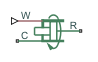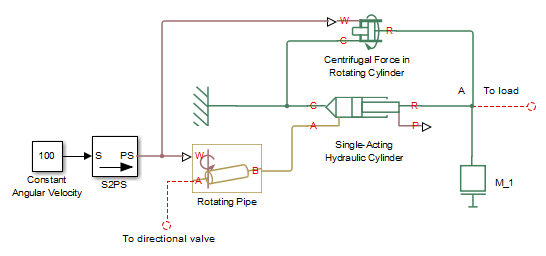# Centrifugal Force in Rotating Cylinder

(To be removed) Centrifugal force in rotating hydraulic cylinders

The Hydraulics (Isothermal) library will be removed in a future release. Use the Isothermal Liquid library instead. (since R2020a)

## Library

Hydraulic Cylinders

•## Description

The Centrifugal Force in Rotating Cylinder block is a building block to be used in hydraulic cylinder models, to account for centrifugal forces exerted by fluid on a piston, if the cylinder rotates about its symmetry axis. Such cylinders are used in control mechanisms of various friction clutches, brakes, square-jaw positive clutches, dog clutches, and so on. No inertial effects are considered in the model. In other words, the angular velocity is assumed to be constant or changing at very low speed.

The centrifugal force is computed with the following equation:

`$F=\frac{\pi \rho {\omega }^{2}}{4}\left({r}_{o}^{4}-{r}_{i}^{4}-2{r}_{p}^{2}\left({r}_{o}^{2}-{r}_{i}^{2}\right)\right)$`

where

 F Centrifugal force ro Piston outer radius ri Piston inner radius rp Fluid entry radius. For design purposes, ${r}_{p}<\sqrt{\left({r}_{o}^{2}+{r}_{i}^{2}\right)/2}$ ρ Fluid density ω Shaft angular velocity

Connections R and C are mechanical translational conserving ports corresponding to the cylinder rod and case, respectively. Connection W is a physical signal port through which shaft angular velocity is imported. The block directionality is adjustable and can be controlled with the Cylinder orientation parameter.

## Basic Assumptions and Limitations

• No inertial effects are considered in the model; that is, the angular velocity of the cylinder is assumed to be constant or changing at very low speed.

• Fluid inertia is not taken into account.

• Fluid compressibility is not taken into account.

## Parameters

The default value is `0.1` m.

The default value is `0.05` m.

The default value is `0.06` m.

Fluid density

The default value is `880` kg/m^3.

Cylinder orientation

Specifies block orientation with respect to the globally assigned positive direction. The block can be installed in two different ways, depending upon whether it exerts force in the positive or in the negative direction. If positive velocity applied at port W exerts force in negative direction, set the parameter to `Acts in negative direction`. The default value is `Acts in positive direction`.

## Examples

The following schematic diagram shows a model of a custom single-acting hydraulic cylinder built of the Single-Acting Hydraulic Cylinder, Rotating Pipe, and Centrifugal Force in Rotating Cylinder blocks.The centrifugal force generated by the Centrifugal Force in Rotating Cylinder block sums up with that of the cylinder at node A. The Rotating Pipe block simulates the pipeline between the cylinder chamber and the channel in the center of a rotating shaft where the cylinder is installed.

## Ports

The block has the following ports:

`R`

Mechanical translational conserving port associated with the cylinder rod.

`C`

Mechanical translational conserving port associated with the cylinder clamping structure.

`W`

Physical signal port providing the angular velocity value.

## Version History

Introduced in R2010a

expand all